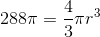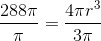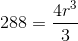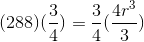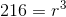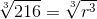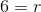# High School Math : How to find the radius of a sphere

## Example Questions

### Example Question #152 : Solid Geometry

Find the radius of a sphere whose surface area is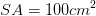.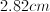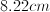Not enough information to solve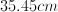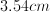Explanation:

We know that the surface area of the spere is.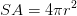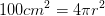Rearrange and solve for.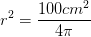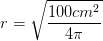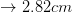### Example Question #153 : Solid Geometry

What is the radius of a sphere that has a surface area of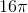?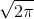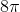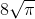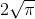Explanation:

The standard equation to find the area of a sphere is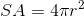wheredenotes the radius. Rearrange this equation in terms of: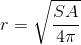To find the answer, substitute the given surface area into this equation and solve for the radius: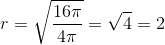### Example Question #154 : Solid Geometry

Given that the volume of a sphere is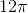, what is the radius?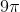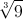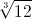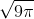Explanation:

The standard equation to find the volume of a sphere is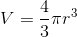wheredenotes the radius. Rearrange this equation in terms of: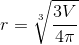Substitute the given volume into this equation and solve for the radius: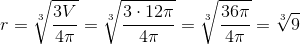### Example Question #155 : Solid Geometry

What is the radius of a sphere with a volume of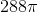?Explanation: